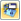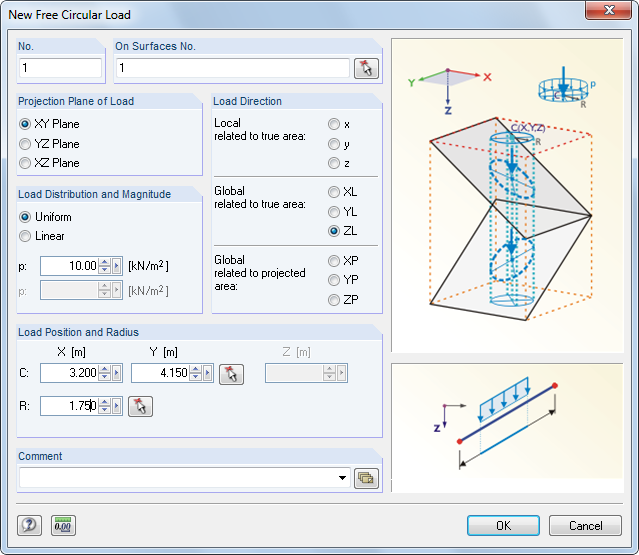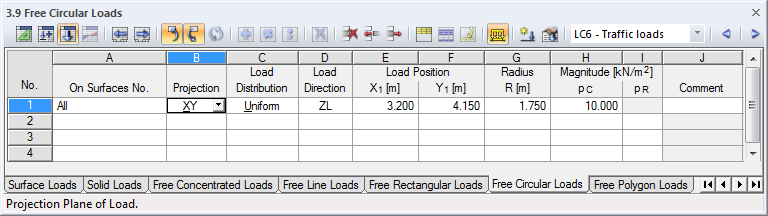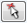# RFEM – Online Manual

Online manuals, introductory examples, tutorials, and other documentation.

# 6.9 Free Circular Loads

General descriptionA free circular load acts as a uniform or linearly variable surface load on a circular, freely definable part of a surface.Figure 6.40 New Free Circular Load dialog boxFigure 6.41 Table 3.9 Free Circular Loads
On Surfaces No.

This text box manages the numbers of the surfaces on which the load acts. You can also select nodes graphically by using.

Starting from the defined Load Position, an imaginary straight line is "set" perpendicular to the projection plane. When the line intersects any of the listed surfaces, the circular load is applied at the point of intersection as the circle center with the radius R. In this way, it is possible to quickly allocate loads of a similar type to several surfaces.

Projection Plane

The load can be projected onto one of the global planes XY, YZ, or XZ. As described above, an imaginary straight line is generated, starting from the load position and running perpendicular to the projection plane. The center of the circular load is assumed wherever the imaginary straight line intersects a surface.

The projection plane may not be perpendicular to a surface on which the load acts: There are no clear points of intersection with the surface.

Load Distribution

Specify whether a uniform or linearly variable force is applied. In the dialog text box below, you can enter one or two numerical values.

Load Direction

The load can act in the direction of the global axes X, Y, Z or the local surface axes x, y, z. The load directions are described in the previous Chapter 6.8.

Load Position

Enter the coordinates of midpoint C of the circle load into the text boxes. In the dialog box, you can also select this point graphically by using thebutton.

Radius

Enter the radius R of the circular area load into the text box or table column. You can also define it graphically in the work window by using thebutton in the dialog box.

Magnitude

In this table column or text box, enter the numerical value of the area load.